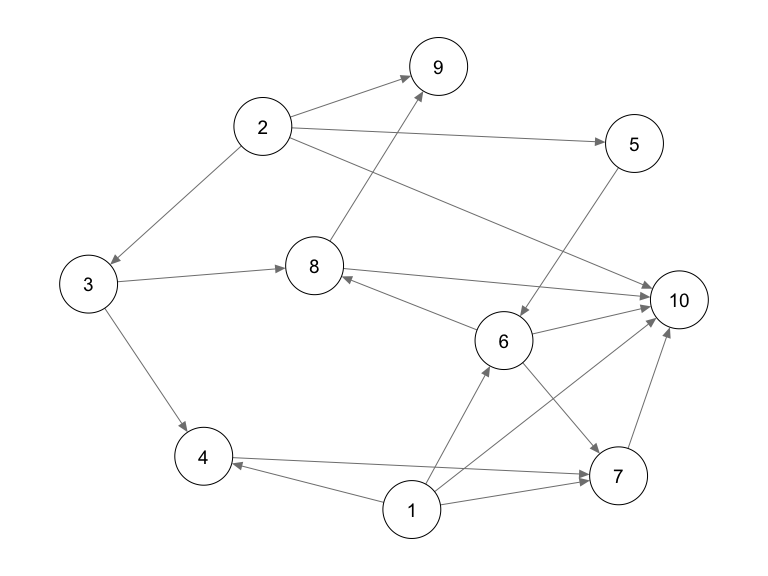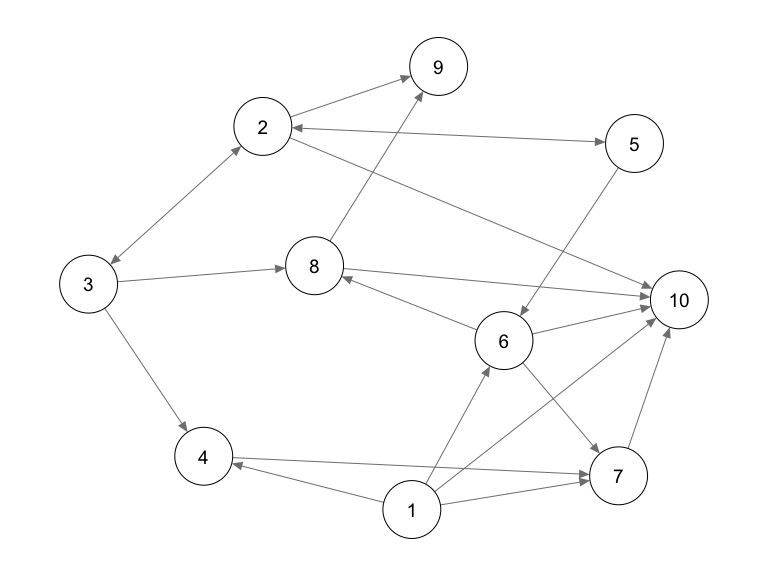eff2: Efficient least squares for total causal effects

We will walk through the use of the package with a built-in example dataset.

library(eff2)
library(qgraph)
data("ex1")

The example dataset is generated from a linear structural equation model (SEM) according to a directed acyclic graph (DAG). There are 10 real-valued variables $$V=\{1,\dots,10\}$$.

q.dag <- qgraph(t(ex1$amat.dag), layout="spring", repulsion=0.5)Note: adjacency matrix in this package follows the convention of package pcalg: • amat[i,j]=0} and amat[j,i]=1 means i->j • amat[i,j]=1 and amat[j,i]=0 means i<-j • amat[i,j]=0 and amat[j,i]=0 means i j • amat[i,j]=1 and amat[j,i]=1 means i--j Hence, for plotting with qgraph, a transpose t() is taken. The observational data is generated from $X_i = \sum_{j} B_{ij} X_j + \varepsilon_i, \quad i \in V$ where $$\{\varepsilon_i: i \in V\}$$ are independent errors. The non-zero pattern of coefficient matrix $$B$$ is encoded by the adjacency matrix of the DAG. That is, $$B_{ij} \neq 0$$ only if $$A_{ij} = 1$$. Total effect For vertices $$i$$ and $$j$$ such that there is a causal path $$i \rightarrow \dots \rightarrow j$$, $$i$$ has a (generically) non-zero total effect $$\tau_{ij}$$ on $$j$$. Given the DAG, the effect can be estimated from data. We will use a simulated dataset that consists of 500 observations. str(ex1$data)
#> 'data.frame':    500 obs. of  10 variables:
#>  $1 : num -0.722 -0.542 -1.493 1.654 0.486 ... #>$ 2 : num  -0.2 0.9172 -2.077 -0.8071 -0.0213 ...
#>  $3 : num -0.853 0.74 -2.306 1.106 -0.581 ... #>$ 4 : num  -1.001 2.282 -0.548 0.959 -1.293 ...
#>  $5 : num -2.07 -1.56 3.21 1.31 -1.04 ... #>$ 6 : num  2.0344 1.6172 0.0598 -2.2497 -3.134 ...
#>  $7 : num 2.2 -3.37 4 -4.08 1.99 ... #>$ 8 : num  0.83 -0.315 1.234 1.796 2.569 ...
#>  $9 : num -1.116 1.507 -0.517 -4.414 -3.573 ... #>$ 10: num  -6.64 -1.62 3.27 8.41 6.03 ...

For example, the total effect from 1 to 10 is estimated as

estimateEffect(ex1$data, 1, 10, ex1$amat.dag)
#>       10
#> 2.005875

Compare this with the true total effect

eff2:::getEffectsFromSEM(ex1$B, 1, 10) # truth #>  2.016003 Here, the effect is in terms of variable 1 is intervened on (point intervention). Similarly, we can estimate the total effect of several variables on a target (joint intervention). For example, consider the total effect of (1,2) on 10: estimateEffect(ex1$data, c(1,2), 10, ex1$amat.dag) #> 1 2 #> 2.0058746 -0.7623894 eff2:::getEffectsFromSEM(ex1$B, c(1,2), 10)   # truth
#>          1          2
#>  2.0160027 -0.7809264

Partial graphical knowledge

Typically, however, the underlying causal DAG is unavailable to us. One can try to estimate such a DAG from observational data. This task is called causal discovery or structure learning; we recommend taking a look at package pcalg, which provides several methods.

Nevertheless, without making additional assumptions, only the Markov equivalence class of the DAG can be recovered. In particular, for certain edges, their directions may not be determined. A Markov equivalence classes (or its further refinements due to background knowledge) is represented by a CPDAG (MPDAG).

qgraph(t(ex1$amat.cpdag), bidirectional=TRUE, layout=q.dag$layout)We can see that the directions of 2--3 and 2--5 are undetermined (drawn as bi-directed above).

We can still estimate total effects based on the graph above. For example, the effect from 1 to 10:

estimateEffect(ex1$data, 1, 10, ex1$amat.cpdag)
#>       10
#> 2.005875
eff2:::getEffectsFromSEM(ex1$B, 1, 10) # truth #>  2.016003 However, because some edges are unoriented, not all total effects can be identified. estimateEffect(ex1$data, c(1,2), 10, ex1$amat.cpdag) #> Error in estimateEffect(ex1$data, c(1, 2), 10, ex1$amat.cpdag): Effect is not identified! An error will be thrown if you try to estimate these unidentified total effects. Function isIdentified can be called to determine if a total effect can be estimated. For example, isIdentified(ex1$amat.cpdag, c(1,2), 10)
#>  FALSE
isIdentified(ex1$amat.cpdag, c(1,6), 10) #>  TRUE and isIdentified(ex1$amat.cpdag, 3, 7)
#>  FALSE
isIdentified(ex1$amat.cpdag, 5, 7) #>  FALSE isIdentified(ex1$amat.cpdag, c(3,5), 7)
#>  TRUE

For statistical inference (e.g., constructing confidence intervals), standard error covariance (asymptotic covariance divided by n) can also be computed by setting bootstrap=TRUE.

result <- estimateEffect(ex1$data, c(3,5), 7, ex1$amat.cpdag, bootstrap=TRUE);
print(result$effect) #> 3 5 #> -1.6393937 0.2026642 # 95% CI print(result$effect - 1.96 * sqrt(diag(result$se.cov))) #> 3 5 #> -1.7614278 0.1590113 print(result$effect + 1.96 * sqrt(diag(result$se.cov))) #> 3 5 #> -1.5173595 0.2463171 # truth eff2:::getEffectsFromSEM(ex1$B, c(3,5), 7)
#>          3          5
#> -1.7215530  0.2285244